# 一、如何判断数据库索引是否生效

• table：顾名思义，显示这一行的数据是关于哪张表的；

• type：这是重要的列，显示连接使用了何种类型。从最好到最差的连接类型为：const、eq_reg、ref、range、indexhe和ALL；

• possible_keys：显示可能应用在这张表中的索引。如果为空，没有可能的索引。可以为相关的域从where语句中选择一个合适的语句；

• key： 实际使用的索引。如果为NULL，则没有使用索引。很少的情况下，MySQL会选择优化不足的索引。这种情况下，可以在Select语句中使用USE INDEX（indexname）来强制使用一个索引或者用IGNORE INDEX（indexname）来强制MySQL忽略索引；

• key_len：使用的索引的长度。在不损失精确性的情况下，长度越短越好；

• ref：显示索引的哪一列被使用了，如果可能的话，是一个常数；

• rows：MySQL认为必须检查的用来返回请求数据的行数；

• Extra：关于MySQL如何解析查询的额外信息。

# 二、哪些场景会造成索引生效

## 1、应尽量避免在 where 子句中使用 != 或 <> 操作符## 2、尽量避免在 where 子句中使用 or 来连接条件## 4、如果列类型是字符串，那一定要在条件中将数据使用引号引用起来，否则不会使用索引；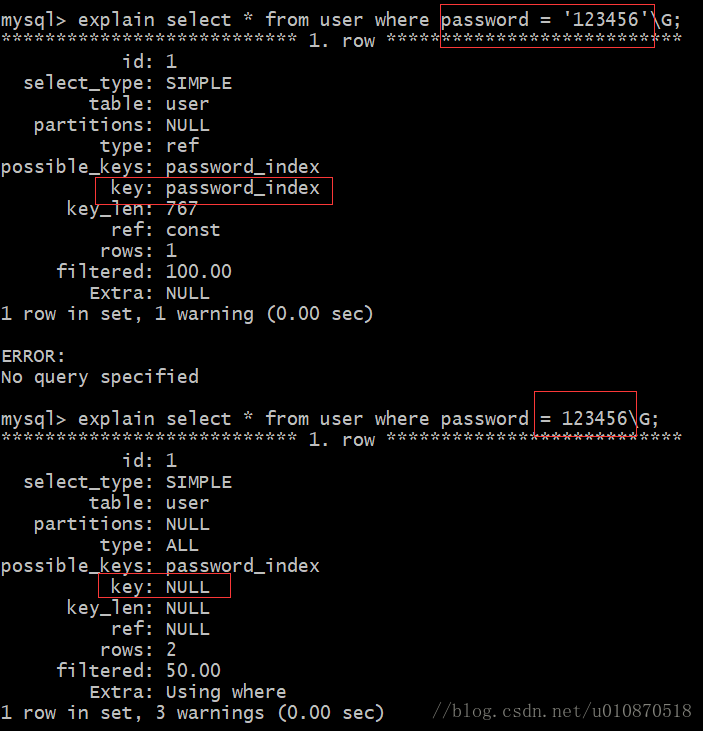## 5、like的模糊查询以 % 开头，索引失效；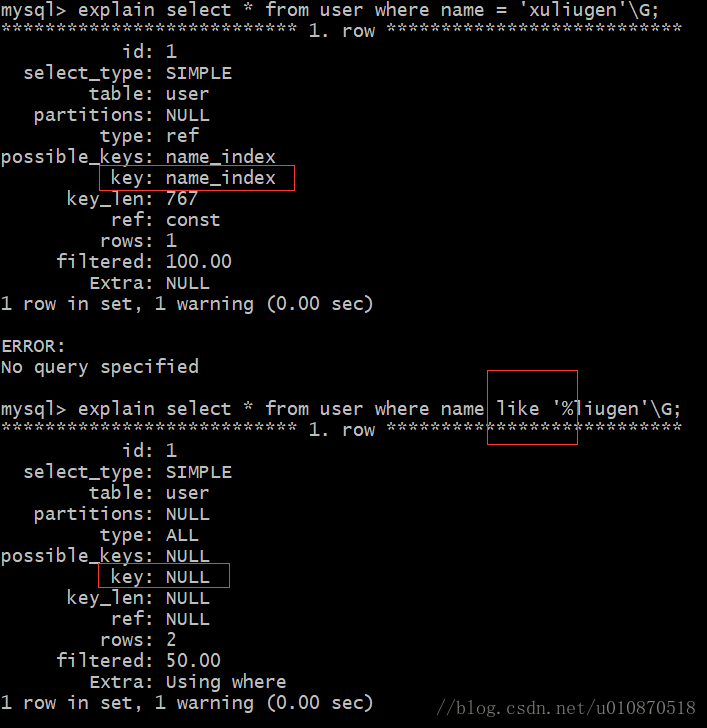但是非前导模糊查询则可以：

`select * from user where name like xuliu%';`

## 6、应尽量在 where 子句中对字段进行表达式操作

`select id from t where num/2 = 100`

`select id from t where num = 100*2；`

## 7、应尽量在 where 子句中对字段进行函数操作

`select id from t where substring(name,1,3) = 'abc' – name;`

`select id from t where name like ‘abc%’`
`select id from t where datediff(day, createdate, '2005-11-30') = 0 – '2005-11-30';`

`select id from t where createdate >= '2005-11-30' and createdate < '2005-12-1';`

# 三、最左前缀原则

（1）如果第一个字段是范围查询需要单独建一个索引；

（2）在创建多列索引时，要根据业务需求，where子句中使用最频繁的一列放在最左边；

`mysql>SELECT `a`,`b`,`c` FROM A WHERE `a`='a1' ; //索引生效 mysql>SELECT `a`,`b`,`c` FROM A WHERE `b`='b2' AND `c`='c2'; //索引失效 mysql>SELECT `a`,`b`,`c` FROM A WHERE `a`='a3' AND `c`='c3'; //索引生效，实际上值使用了索引a`

## 3.1、三个字段联合索引测试：联合索引的顺序为：sex，age，name

`SELECT * FROM user where sex="3"; #使用索引 SELECT * FROM user where age="4"; #未使用索引 SELECT * FROM user where name="2"; #未使用索引 SELECT * FROM user where sex="2" and age="3"; #使用索引 SELECT * FROM user where sex="2" and age="3" and name="4"; #使用索引 SELECT * FROM user where age="3" and name="4"; #未使用索引 SELECT * FROM user where sex="2" and name="4"; #使用索引 #这个在3.2最后边解释，为什么 explain SELECT * FROM index_demo.user where age="2" and sex="3"; #使用索引`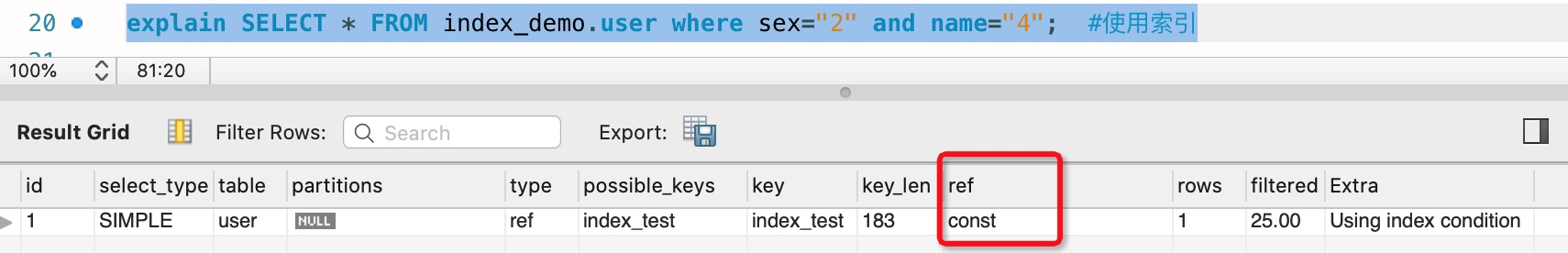和where sex=“2” and age=“3” and name="4"的区别：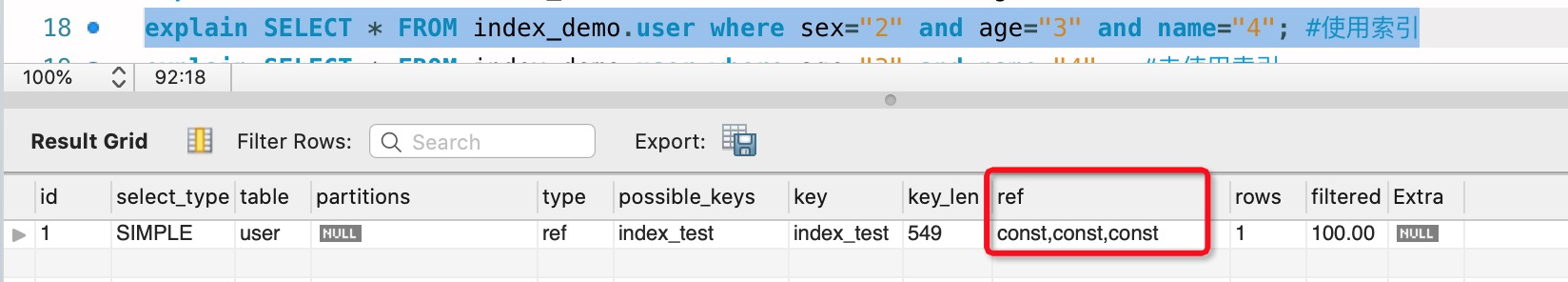## 3.2、如果索引字段有两个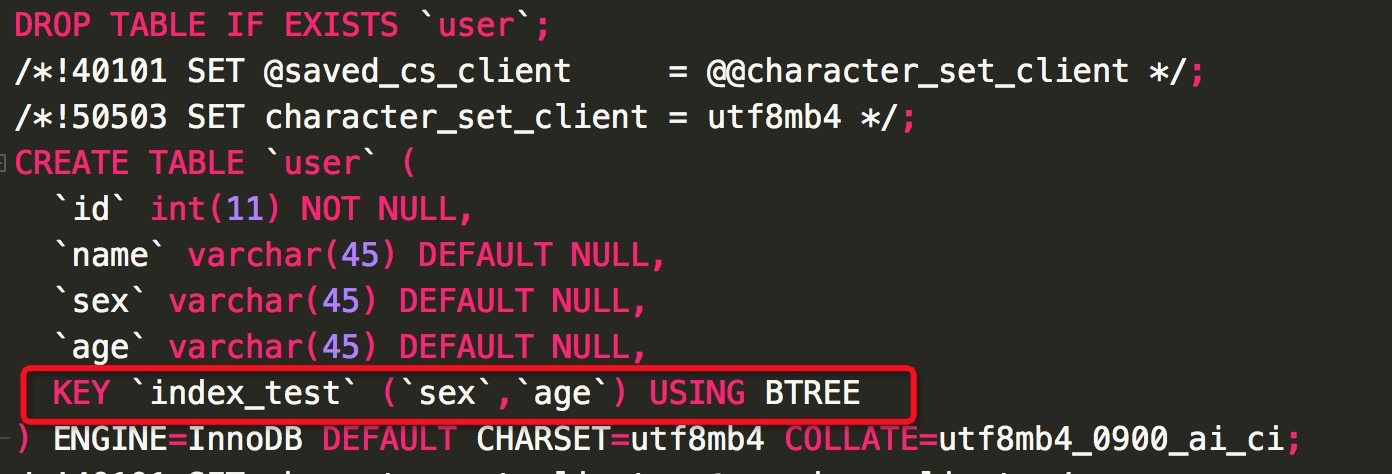`explain SELECT * FROM index_demo.user where sex="3"; #使用索引 explain SELECT * FROM index_demo.user where age="4"; #未使用索引 explain SELECT * FROM index_demo.user where sex="2" and age="3"; #使用索引 explain SELECT * FROM index_demo.user where age="3" and sex="4"; #使用索引`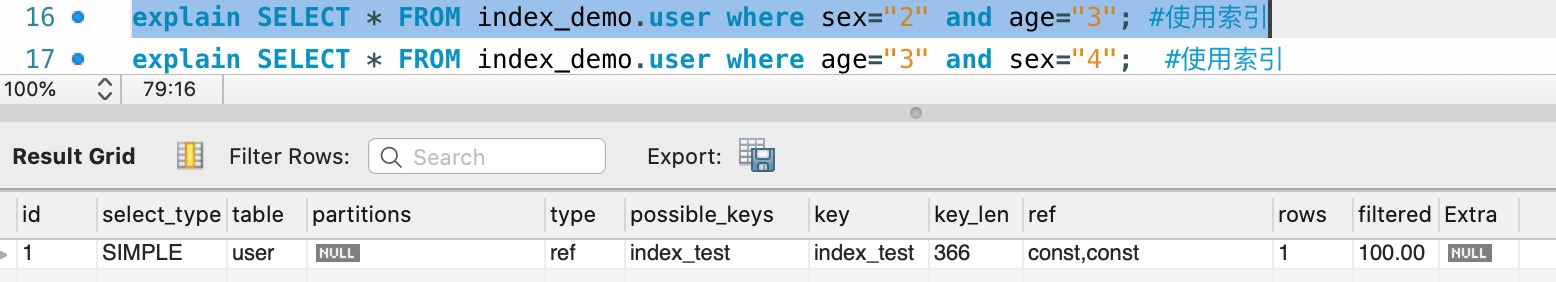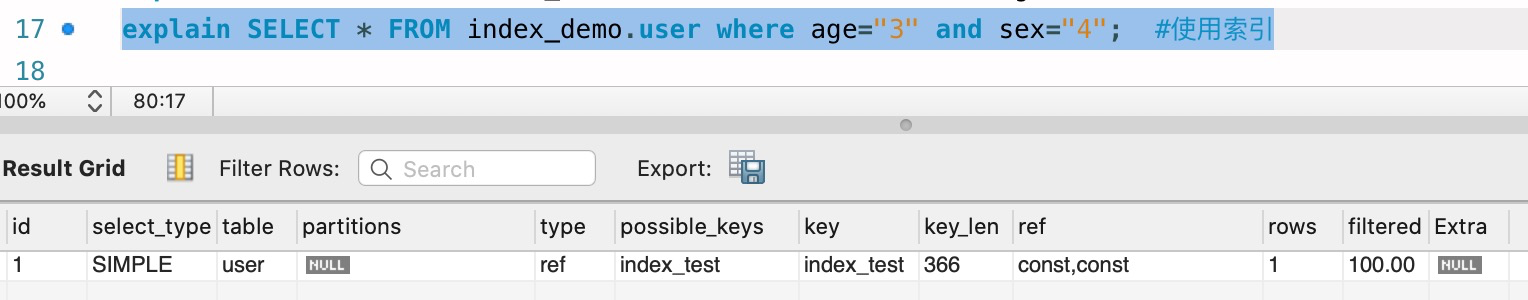where sex=“2” and age=“3”;和where sex=“2” and age=“3”;

`explain SELECT * FROM index_demo.user where age="2" and sex="3"; #使用索引`

1、http://blog.csdn.net/qq_33774822/article/details/61197420